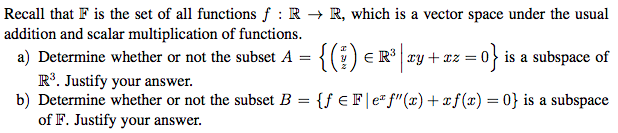# Question Solved1 AnswerRecall that F is the set of all functions f :R + R, which is a vector space under the usual addition and scalar multiplication of functions. a) Determine whether or not the subset A = { =0} is a subspace of R3. Justify your answer. b) Determine whether or not the subset B = {f EF|ef"(2) +2f(0) = 0) is a subspace of F. Justify your answer.ZFKBVS The Asker · Other MathematicsTranscribed Image Text: Recall that F is the set of all functions f :R + R, which is a vector space under the usual addition and scalar multiplication of functions. a) Determine whether or not the subset A = { =0} is a subspace of R3. Justify your answer. b) Determine whether or not the subset B = {f EF|ef"(2) +2f(0) = 0) is a subspace of F. Justify your answer.
More
Transcribed Image Text: Recall that F is the set of all functions f :R + R, which is a vector space under the usual addition and scalar multiplication of functions. a) Determine whether or not the subset A = { =0} is a subspace of R3. Justify your answer. b) Determine whether or not the subset B = {f EF|ef"(2) +2f(0) = 0) is a subspace of F. Justify your answer.